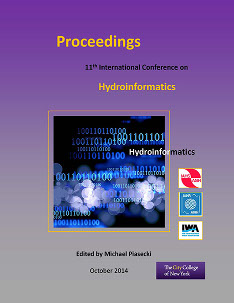## International Conference on Hydroinformatics

Presentation

8-1-2014

#### Abstract

Infiltration of water in the soil is a key process in the hydrological cycle and its understanding is paramount for students of any course related to Hydrology. Different equations exist, which, in function of boundary conditions and the level of detail required in any particular case can become complex to solve in an analytical way. Water movement in the soil can be described by the Richards equation, but its solution requires numerical methods. Therefore, amongst the most used ways of calculating water infiltration are the Green and Ampt method, Horton’s equation, Philip’s equation and the Curve Number method. In all these equations, different soil parameters intervene that can be measured in the field or estimated from soil properties. The understanding of the physical meaning of these parameters, their relative importance and of the differences between the abovementioned methods is important for students. Infiltration measurements in the field or lab are time-consuming. We therefore developed a tool that helps the students to calculate infiltration in a step-by-step way, and which helps to easily change the input parameter values so as to understand the importance of the different variables. This virtual lab environment was coded in Matlab and used for the first time during the academic year 2013-2014. In this study, we present the main characteristics of this didactic software tool and present an evaluation of its usefulness by students.